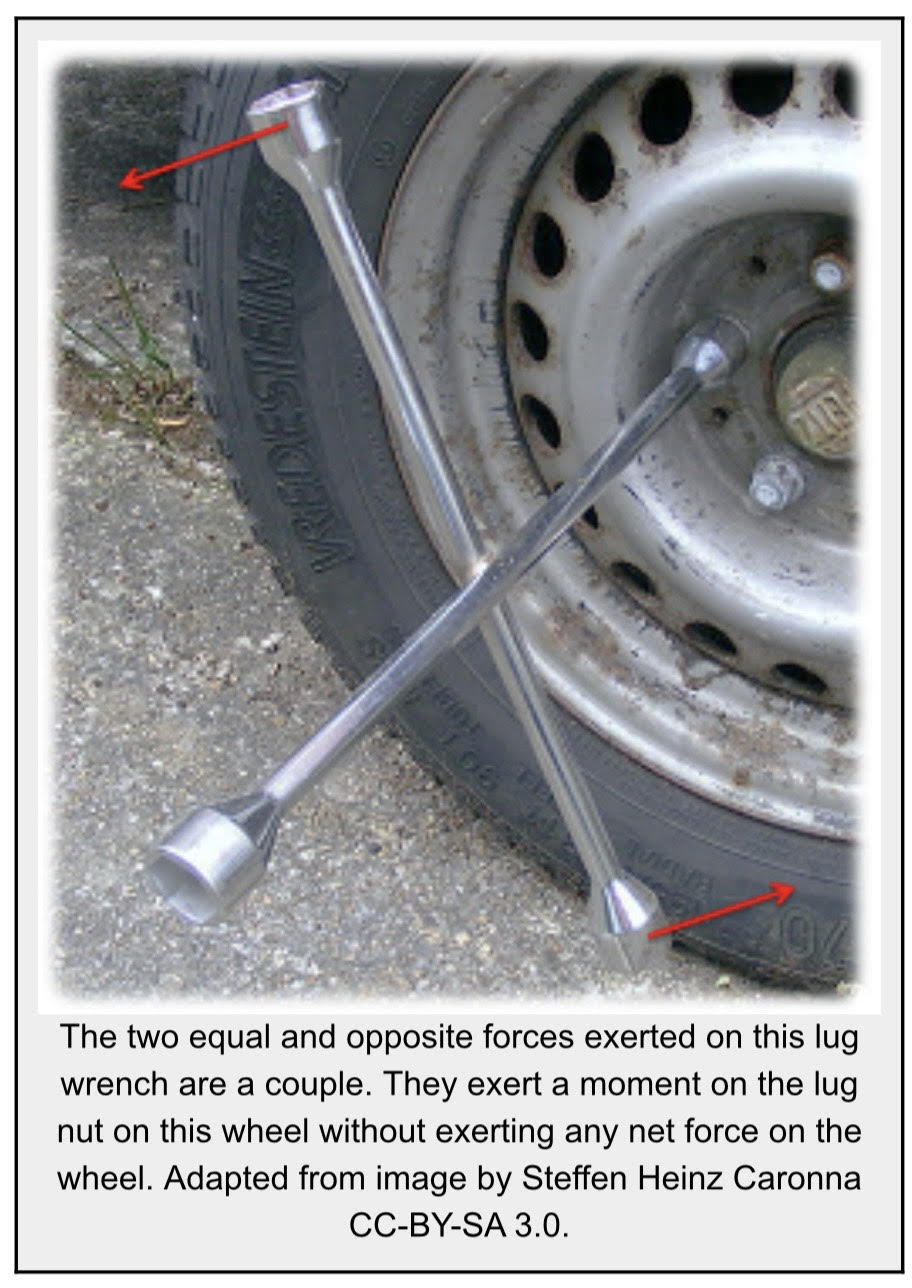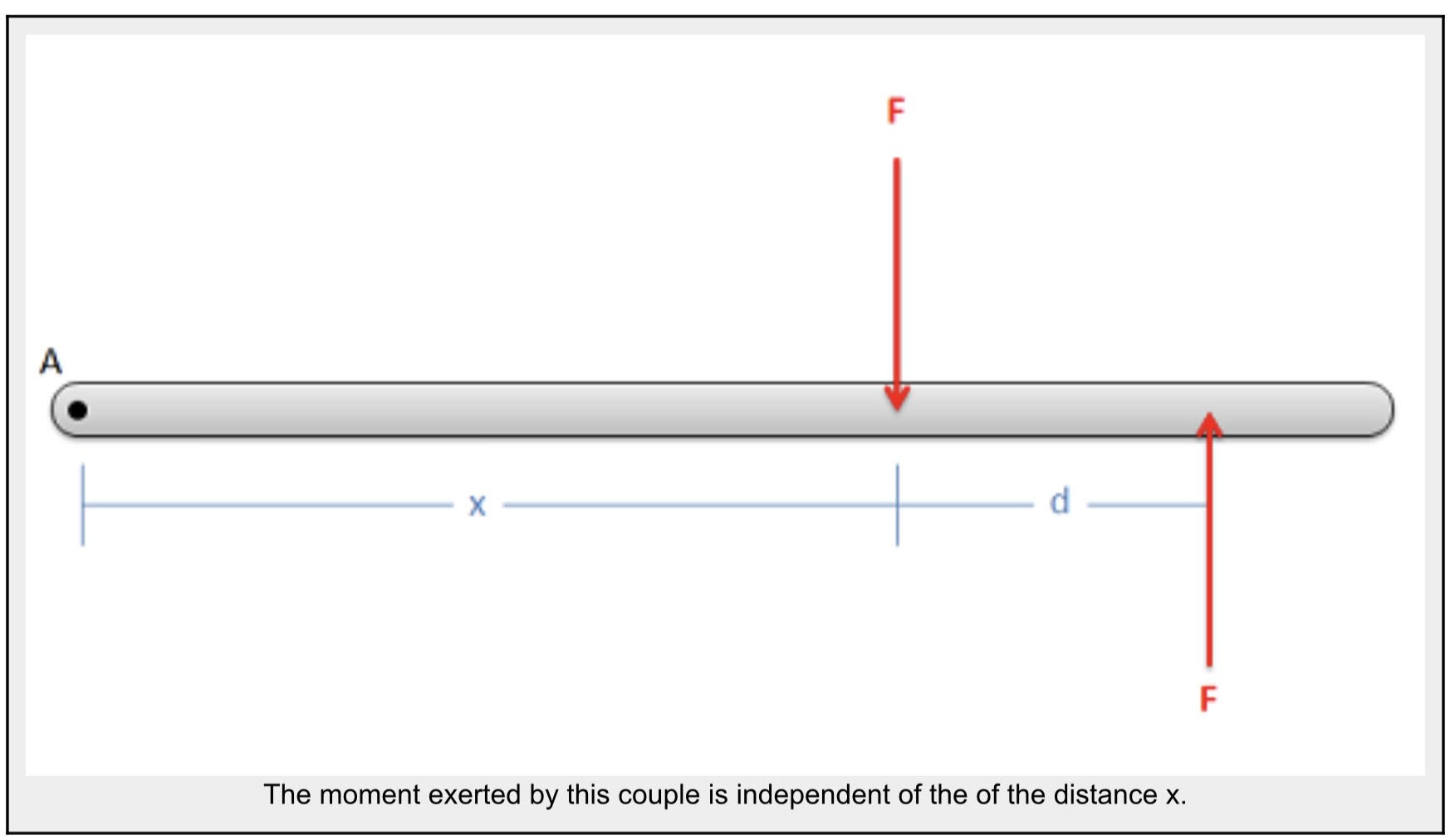Chapter 3: Rigid Body Basics

# 3.2 Couples

couple is a set of equal and opposite forces that exerts a net moment on an object but no net force. Because the couple exerts a net moment without exerting a net force, couples are also sometimes called pure moments.The moment exerted by a couple also differs from the moment exerted by a single force in that it is independent of the location you are taking the moment about. In the example below we have a couple acting on a beam. Each force has a magnitude F and the distance between the two forces is d.Now we have some point A, which is distance x from the first of the two forces. If we take the moment of each force about point A, and then add these moments together for the net moment about point A we are left with the following formula.

$$M=-(F\ast x)+(F\ast(x+d))$$

If we rearrange and simplify the formula above, we can see that the variable x actually disappears from the equation, leaving the net moment equal to the magnitude of the forces (F) times the distance between the two forces (d).

$$M=-(F\ast x)+(F\ast x)+(F\ast d)\\\\M=(F\ast d)$$

This means that no matter what value of x we have, the magnitude of the moment exerted by the couple will be the same. The magnitude of the moment due to the couple is independent of the location we are taking the moment about. This will also work in two or three dimensions as well. The magnitude of the moment due to a couple will always be equal to the magnitude of the forces times the perpendicular distance between the two forces.

Source: Engineering Mechanics, Jacob Moore et al., http://mechanicsmap.psu.edu/websites/3_equilibrium_rigid_body/3-3_couples/couples.html

Key Takeaways

Basically: Couples are made from two forces in opposite directions that create a moment around an axis

Application: Turning the steering wheel of your car, you push one hand up and the other down to turn the wheel. To calculate the size of the couple, you multiply the force exerted by the distance between your hands (the diameter of the wheel).

Looking Ahead: While moments are more common in Ch 4 rigid body equations, it’s important to know what couples are and how to find them.